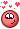## Recommended Posts

Hi,

Is there like a Mask by Fresnel much the same way as Mask by Attribute?
Basically, like Fresnel in shading but instead on rendering, I want to mask all the sides of the geometry (i.e. result of the Fresnel)?

Is this possible? I understand there is Fresnel node but I can't use it on the SOP context only on the mat context.

Edited by bentraje
##### Share on other sites

The Measure SOP can calculate the curvature of geometry. Try using the result of that node.

##### Share on other sites

Hi @Atom

Thanks for the response. But the curvature is for concavity/convexity/. It's those on the crevices of the geo.
The fresnel is on the edges of the geo depending on the viewing angle of the camera.

##### Share on other sites

```vector campos = chv("campos");
vector lgtpos = chv("lgtpos");
float rough = chf("rough");
vector wi = normalize(lgtpos - @P);
vector wo = normalize(campos - @P);
vector h = normalize(wi+wo);

float lambert = max(dot(@N, wi), 0);

float D_GGX_TR(vector N;vector H;float a)
{
float a2     = a*a;
float NdotH  = max(dot(N, H), 0.0);
float NdotH2 = NdotH*NdotH;

float nom    = a2;
float denom  = (NdotH2 * (a2 - 1.0) + 1.0);
denom        = PI * denom * denom;

return nom / denom;
}

float GeometrySchlickGGX(float NdotV; float k)
{
float nom   = NdotV;
float denom = NdotV * (1.0 - k) + k;

return nom / denom;
}

float GeometrySmith(vector N; vector V;vector L; float k)
{
float NdotV = max(dot(N, V), 0.0);
float NdotL = max(dot(N, L), 0.0);
float ggx1 = GeometrySchlickGGX(NdotV, k);
float ggx2 = GeometrySchlickGGX(NdotL, k);
return ggx1 * ggx2;
}
float kdirect(float rough){
return  pow(rough,2)  / 2.0f ;
}

vector fresnelSchlick(float cosTheta; vector F0)
{
return F0 + (1.0 - F0) * pow(1.0 - cosTheta, 5.0);
}

vector rough_tex = texture(chs("image"),@uv.x, @uv.y);
vector diff = texture(chs("diff"),@uv.x, @uv.y);

float ggx_tr = D_GGX_TR(@N,h,rough_tex.x );
float ggx_trbase = D_GGX_TR(@N,h,rough );

float k = kdirect(rough_tex.x);
float ggx_smith = GeometrySmith(@N, wo, wi, k);
ggx_smith = max(ggx_smith , 0.0);
//@Cd = diff + ggx_smith * ggx_tr ;

vector fresnelv =  fresnelSchlick(dot(@N,wo), chf("fresnel" ));

@Cd = diff*.2 + ggx_tr* ggx_smith * fresnelv;```

maybe someone can explain and make this in Real Scene@bentraje

Edited by Librarian
•1
##### Share on other sites

You can achieve this by writing this sort of code inside a Primitive Wrangle:

```string cam = chs("cam");
float near = 0;
float far = -0.01;

vector pnear = fromNDC ( cam, set ( 0.5, 0.5, near ) );
vector pfar = fromNDC ( cam, set ( 0.5, 0.5, far ) );
vector dir = -normalize ( pfar - pnear );

vector n = normalize ( primuv ( 0, "N", @primnum, 0.5 ) );
if ( dot ( n, dir ) < ch("threshold") )
i@group_fresnel = 1;```•4
##### Share on other sites

Thanks for the responses.

@Librarian
I couldn't quite directly use it since I guess the code is for the mat context.
That said I think I get the overall logic. I think its doing Method #2 in the code below.

@animatrix
The code works as expected.

==========

Also just for reference. People from slack group also helped me. Here are the results.
Same goal just different execution.

Method 1 (from bcarmeli)

```vector @cdir;
vector @raydir;
matrix camMatrix = optransform(chs("camera"));
//get a matrix with the camera's transforms.
@cdir = cracktransform(0, 0, 0, {0,0,0}, camMatrix);
//extract out the camera position as a vector
@raydir = normalize(@P-@cdir);
//get a vector to project pointo from camera

f@facing_ratio = fit11(dot(@N, @raydir),0,1);

if(@facing_ratio>chf("threshold")){
@group_mygroup = 1;
}```

Method 2   (from David Torno)

```vector cam = getpointbbox_center(1);

//Option 1: Get dir per point
vector dir = normalize(@P - cam);

//Option 2: Get single point centroid
//vector c = getpointbbox_center(0);
//vector dir = normalize(@P - c);

float d = fit11(dot(@N, dir), 0, 1);

if(d > chf("threshold"))i@group_mygroup =1;```

Will close this thread now. Thanks for the help!

•2
##### Share on other sites

@bentraje
just use groupfromattribboundary SOP on PRIM 'Cd' endless control.

•2

## Join the conversation

You can post now and register later. If you have an account, sign in now to post with your account.
Note: Your post will require moderator approval before it will be visible.×   Pasted as rich text.   Paste as plain text instead

Only 75 emoji are allowed.

×   Your previous content has been restored.   Clear editor

×   You cannot paste images directly. Upload or insert images from URL.

×
×